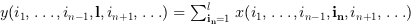Scilab-Branch-5.3-GIT
Change language to:
Français - 日本語 - Português

See the recommended documentation of this function

# cumsum

cumulative sum of array elements

### Calling Sequence

```y=cumsum(x)
y=cumsum(x,orientation)
y=cumsum(x,outtype)
y=cumsum(x,orientation,outtype)```

### Arguments

x

an array of reals, complex, booleans, polynomials or rational fractions.

orientation

This argument can be

• either a string with possible values `"*"`, `"r"`, `"c"` or `"m"`

• or a number with positive integer value

outtype

a string with possible values `"native"` or `"double"`.

y

scalar or array

### Description

For an array `x`, `y=cumsum(x)` returns in the scalar `y` the cumulative sum of all the elements of `x`.

`y=cumsum(x,orientation)` returns in `y` the cumulative sum of `x` along the dimension given by `orientation`:

• if `orientation` is equal to 1 or "r" thenor• Si `orientation` est égal à 2 ou "c" alors:or• if `orientation` is equal to n then• `y=cumsum(x,"*")` is equivalent to `y=cumsum(x)`

• `y=cumsum(x,"m")` is equivalent to `y=cumsum(x,orientation)` where `orientation` is the index of the first dimension of `x` that is greater than 1. This option is used for Matlab compatibility.

The `outtype` argument rules the way the summation is done:

• For arrays of floats, of polynomials, of rational fractions, the evaluation is always done using floating points computations. The `"double"` or `"native"` options are equivalent.

• For arrays of integers,

if `outtype="native"` the evaluation is done using integer computations (modulo 2^b, where b is the number of bits used),

if `outtype="double"` the evaluation is done using floating point computations.

The default value is `outtype="native"`.

• For arrays of booleans,

if `outtype="native"` the evaluation is done using boolean computations ( + is replaced by |),

if `outtype="double"` the evaluation is done using floating point computations (%t values are replaced by 1 and %f values by 0).

The default value is `outtype="double"`.

### Remark

This function applies, with identical rules to sparse matrices and hypermatrices.

### Examples

```A=[1,2;3,4];
cumsum(A)
cumsum(A,1)

I=uint8([2 95 103;254 9 0])
cumsum(I) //native evaluation
cumsum(I,"double")
cumsum(I,2,"double")

s=poly(0,"s");
P=[s,%i+s;s^2,1];
cumsum(P),
cumsum(P,2)

B=[%t %t %f %f];
cumsum(B) //evaluation in float
cumsum(B,"native") //similar to or(B)```